Courses

# VITEEE Maths Test - 8

## 40 Questions MCQ Test VITEEE: Subject Wise and Full Length MOCK Tests | VITEEE Maths Test - 8

Description
This mock test of VITEEE Maths Test - 8 for JEE helps you for every JEE entrance exam. This contains 40 Multiple Choice Questions for JEE VITEEE Maths Test - 8 (mcq) to study with solutions a complete question bank. The solved questions answers in this VITEEE Maths Test - 8 quiz give you a good mix of easy questions and tough questions. JEE students definitely take this VITEEE Maths Test - 8 exercise for a better result in the exam. You can find other VITEEE Maths Test - 8 extra questions, long questions & short questions for JEE on EduRev as well by searching above.
QUESTION: 1

Solution:
QUESTION: 2

Solution:
QUESTION: 3

### If z = [(1-i√3)/(1+i√3)], then arg (z) =

Solution:
QUESTION: 4

If ω is a complex cube root of unity, then 225 + (3ω+8ω2)2+3ω2+8ω)2=

Solution:
QUESTION: 5

If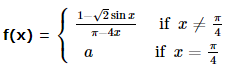is continuous at π/4 , then a =

Solution:
QUESTION: 6Solution:
QUESTION: 7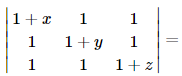Solution:
QUESTION: 8

In determinant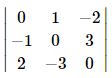radio of the cofactor of -3 and subdeterminant is

Solution:
QUESTION: 9

Let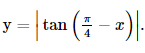Then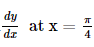is

Solution: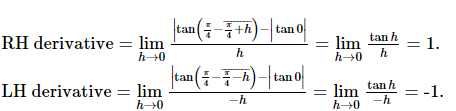QUESTION: 10

The degree and order of the differential equation of the family of all parabolas whose axis is x-axis are respectively

Solution:
QUESTION: 11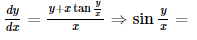Solution:
QUESTION: 12

The order and degree of the differential equation d2y/dx2 + (dy/dx)1/3 + x1=0 are respectively

Solution:
QUESTION: 13

Solution of cos x dy/dx+y sin x =1 is

Solution:
QUESTION: 14

The differential of sin-1[(1-x)/(1+x)] w.r.t. √x is equal to

Solution:
QUESTION: 15

If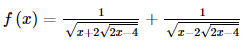for x > 2, then f 11

=

Solution:
QUESTION: 16

If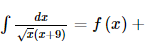constant , then f (x)   =

Solution:
QUESTION: 17

If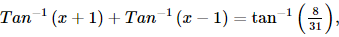then x =

Solution:
QUESTION: 18

∫[(x2+1)/(x4-x2+1)] dx =

Solution:
QUESTION: 19

The value of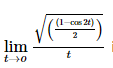is

Solution:
QUESTION: 20

A function f(x) is defined as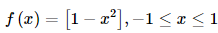, where [x] denotes the greatest integer not exceeding x. The function f(x) is discontinuous at x = 0 because

Solution:
QUESTION: 21

Negation of "Sohan is in class X or Rani is in class XII" is

Solution:
QUESTION: 22

If A is a non-singular, then adj A is

Solution:
QUESTION: 23

If A is a square matrix of order 3, then which of the following is correct.(where I is a unit matrix)

Solution:
QUESTION: 24

The number of 4-digit even numbers that can be formed using 0, 1, 2, 3, 4, 5, 6 without repetition is

Solution:

Each even number must have 0, 2, 4 or 6 in is units place
Here total number of digits = 7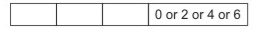When 0 occurs at units place there is no restriction on other places and when 2 or 4 occurs at units place there is restriction on thousands' place as 0 can not be put at thousands' place
Case I When 0 occurs at units place: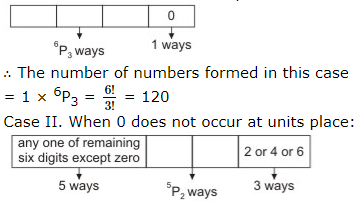The units' place can be filled up by any one of the three digits 2, 4 and 6 in 3 ways
∴ The number of numbers formed in this case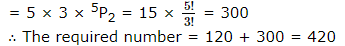QUESTION: 25

A,B,C in order toss a coin. The first one to throw a head wins. Assuming the game continues indefinitely, their respective chances of winning the game are

Solution:
QUESTION: 26

Three letters are written to different persons and addresses on three envelopes are also written. Without looking at the addresses, the probability that the letters go into the right envelope, is

Solution:
QUESTION: 27

Give two independent events A and B such that P(A) = 0.30 and P(B) = 0.60. Prob of getting neither A nor B is

Solution:
QUESTION: 28

In a triangle ABC, A = 300, b = 8, a = 6, then B = sin-1 x where x =

Solution:
QUESTION: 29

The number of terms common to two A.P.'s 3, 7, 11, ..., 407 and 2, 9, 16,...., 709 is

Solution:
QUESTION: 30

The first of the two samples has 63 items with mean 27.6 and standard deviation 7.1. If the whole group has 89 items with mean 25.1 and standard deviation 7.8, the standard deviation of the second group is

Solution:
QUESTION: 31

The quartile deviation of daily wages (in Rs.) of 7 persons given below:
12, 7, 15, 10, 17, 17, 25 is

Solution:
QUESTION: 32

In ∆ABC angle A is defined by 5 cos A + 3 = 0, the equation whose roots are sinA and tanA, is

Solution:
QUESTION: 33

The roots of the equation x3 + x2 + x + 1 = 0 are___

Solution: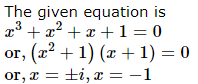QUESTION: 34

The shortest distance from the point (1, 2, -1) to the surface of the sphere x2 + y2 + z2 = 24 is

Solution:
QUESTION: 35

A st. line which makes an angle of 600 with each of y and z axes, inclines with x-axis at an angle

Solution:
QUESTION: 36

If sin2θ-2cosθ+(1/4)=0, the general value of θ is

Solution:
QUESTION: 37

The locus of a point P(α, β) moving under the condition that the line y = αx + β is a tangent to the hyperbola (x2/a) - (y2/b2) = 1 is

Solution:
QUESTION: 38

The length of the tangent from the point (5, 4) to the circle
x2 + y2 + 2x - 6y = 6 is

Solution:
QUESTION: 39

If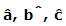are three unit vectors such that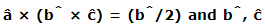are non collinear, then the angles that â makes with b̂ and ĉ respectively are

Solution:
QUESTION: 40

In a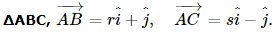If the area of triangle is of unit magnitude, then

Solution: